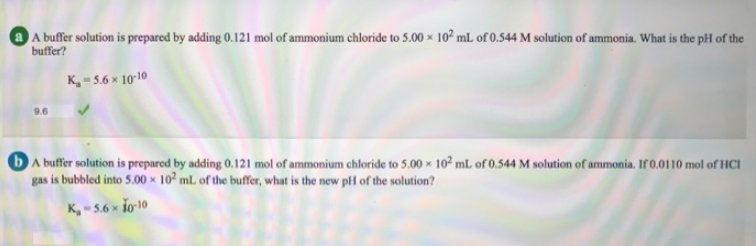# Problem: A buffer solution is prepared by adding 0.121 mol of ammonium chloride to 5.00 times 10^2 mL of 0.544 M solution of ammonia. What is the pH of the buffer? Ka = 5.6 times 10^-10 A buffer solution is prepared by adding 0.121 mol of ammonium chloride to 5.00 times 10^2 mL of 0.544 M solution of ammonia. If 0.0110 mol of NCI gas is bubbled into 5.00 times 10^2 mL of the buffer, what is the new pH of the solution? Ka = 5.6 times 10^-10

###### FREE Expert Solution
79% (118 ratings)###### Problem Details

A buffer solution is prepared by adding 0.121 mol of ammonium chloride to 5.00 times 10^2 mL of 0.544 M solution of ammonia. What is the pH of the buffer? Ka = 5.6 times 10^-10 A buffer solution is prepared by adding 0.121 mol of ammonium chloride to 5.00 times 10^2 mL of 0.544 M solution of ammonia. If 0.0110 mol of NCI gas is bubbled into 5.00 times 10^2 mL of the buffer, what is the new pH of the solution? Ka = 5.6 times 10^-10